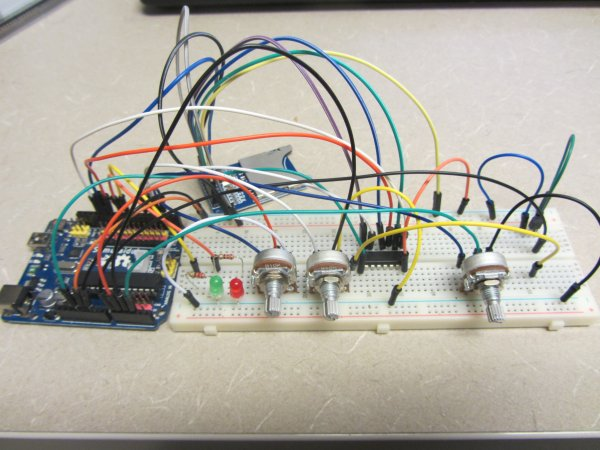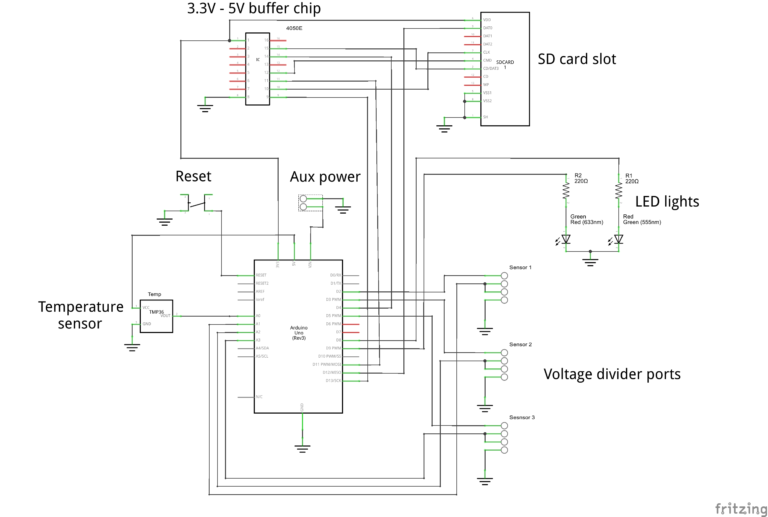# Arduino Resistance Data LoggerI was working on a project were I needed a cheap and efficient way to log the changing resistance of a sensor while simultaneously logging the temperature. This project is similar in many respects to the other data loggers on my site. Three prototypes have been built with the third one being a PCB. I have found this design to work extremely well for my needs.The resistance measurement principle is based on a simple resistive divider. An unknown resistor (the sensor in my case) is attached to the top leg of the divider, while a known resistor (reference resistor) is attached to the bottom leg of the resistor. The voltage between the two resistors (V_out) is than measured and stored on a SD card. The stored voltage data is than manipulated in MATLAB and converted into a resistance value based on the size of the reference resistor. The SD card is able to hold both the collected data from the Arduino, and the MATLAB script used for converting the data into resistance. Keeping the MATLAB script on the SD card allows for easy and convenient data processing without having keep a data processing script in a separate location.Read more: Arduino Resistance Data Logger